# Mobius Volume Waves for ThinkorSwim

#### MerryDay

Staff member
Staff
VIP
Mobius Volume Waves found it on JohnnyQuotron's OneNote
Mobius's description:
A very simple Volume study based on accumulation or distribution. AKA Volume Waves.

After much research, I conclude there is no syntax that will give CONSISTENT results when:
• scanning
• painting candles
This is due to the multiple levels of recursion built-into the definition of the waves.
The Volume Waves study was created to give a clear visual representation of how volume is driving price action.

To looking for the flip between buying pressure and selling pressure, this is not the correct tool.
These threads provide many variations for determining that: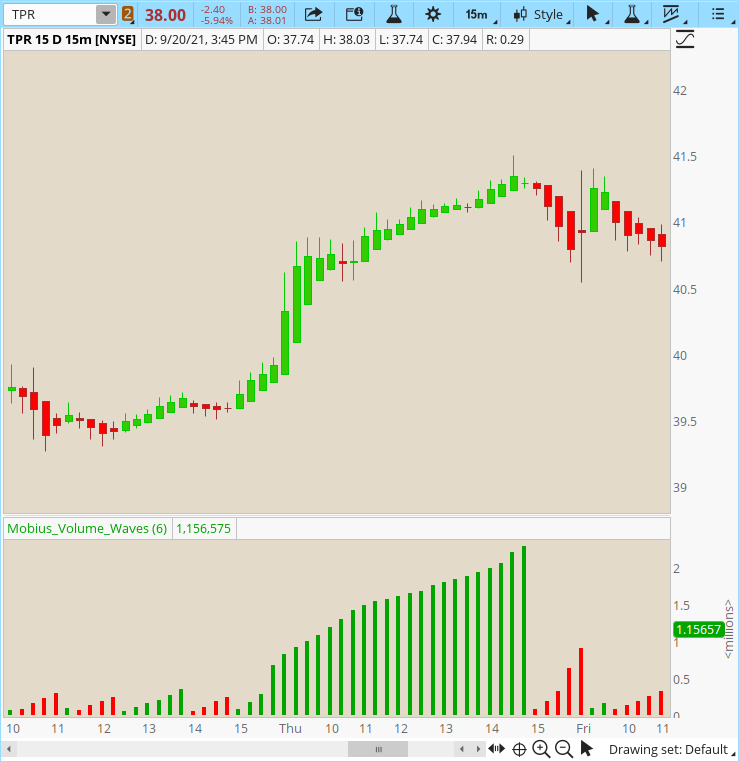Chart:

Ruby:
``````# Mobius Volume Wave
# V01.2020
# Plots Volume Waves based on trend.

declare lower;

input n = 6;

DefineGlobalColor("LabelGreen",  CreateColor(0, 165, 0)) ;
def v = volume;
def accumulation = isAscending(HL2, n);
def distribution = isDescending(HL2, n);

def w = if accumulation and !accumulation
then v
else if distribution and !distribution
then v
else w + v;
plot waves = w;
waves.SetPaintingStrategy(PaintingStrategy.Histogram);
waves.AssignValueColor(if accumulation
then GlobalColor("LabelGreen")
else if distribution
then color.red
else color.yellow);
# End Code Mobius Volume Waves``````

Last edited:
••wvie2021, EvilSurgeon, wtf_dude and 2 others

#### MerryDay

Staff member
Staff
VIP
Mobius Supertrend Volume Waves also from on JohnnyQuotron's OneNote
This indicator has no correlation and it is not related AT ALL with the Mobius SuperTrend.​
The Supertrend Volume Waves is the exact same as the Volume Waves except it has an additional plot:
plot avg = (HighestAll(w1/2)+highestAll(w2/2))/n ;​
It plots the highest average of the bearish and bullish waves. Waves that are above the average plot are said to be on a super trend.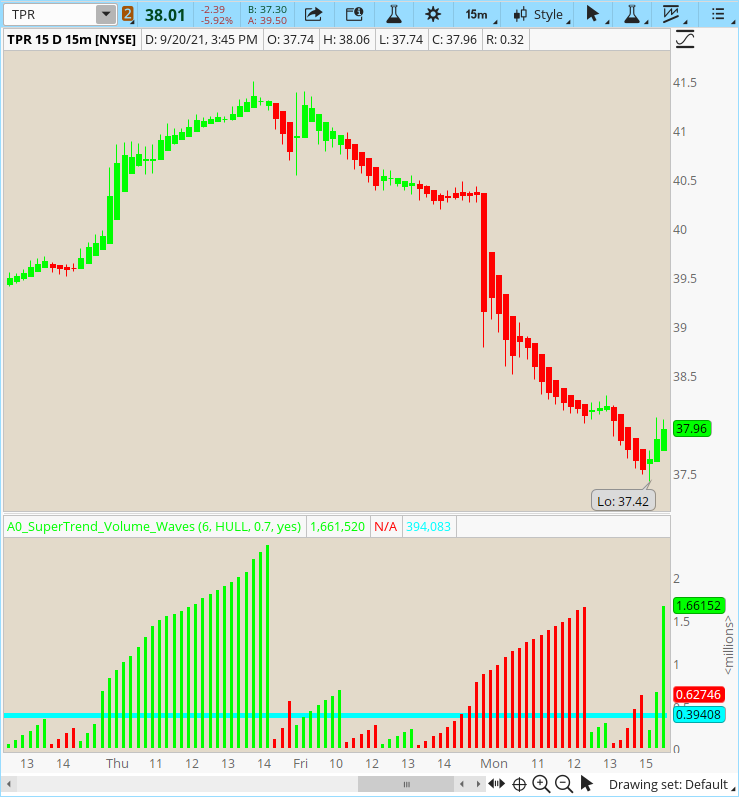Ruby:
``````# Mobius Supertrend Volume Waves
# V01.2020
# Plots Volume Waves based on trend.

declare lower;

input n = 6;
input AvgType = AverageType.HULL;
input ATRmult = .7;
input ColorCandles = no;
def h = high;
def l = low;
def c = close;
def v = volume;
DefineGlobalColor("Ascending", Color.GREEN);
DefineGlobalColor("descending", Color.RED);
DefineGlobalColor("avg", Color.CYAN);
def ATR = MovingAverage(AvgType, TrueRange(h, c, l), n);
def DN = HL2 + (AtrMult * ATR);
def UP = HL2 + (-AtrMult * ATR);
def cond = if c < cond
then DN
else UP;
def accumulation = cond == UP;
def distribution = cond == DN;
def w1 = if accumulation and !accumulation
then v
else if accumulation
then w1 + v
else Double.NaN;
def w2 = if distribution and !distribution
then v
else if distribution
then w2 + v
else Double.NaN;
plot waves1 = w1;
waves1.SetPaintingStrategy(PaintingStrategy.HISTOGRAM);
waves1.SetDefaultColor(GlobalColor("Ascending"));
plot waves2 = w2;
waves2.SetPaintingStrategy(PaintingStrategy.HISTOGRAM);
waves2.SetDefaultColor(GlobalColor("Descending"));
def SD = StDevAll(v);
plot avg = (HighestAll(w1/2)+highestAll(w2/2))/n ;
avg.SetDefaultColor(GlobalColor("avg"));
AssignPriceColor(if ColorCandles and cond == dn
then color.red
else color.green);
[INDENT]# End Code``````
[/INDENT]

Last edited:

#### MerryDay

Staff member
Staff
VIP
The thired and last of Mobius's Volume Waves:
Mobius Supertrend Volume Waves with Range Labels
This indicator has no correlation and it is not related AT ALL with the Mobius SuperTrend.​
Mobius's description:
Some additions that I see could be useful. A typical range for a wave.
These simple statistics labels tabulate the total number of bars in your data set as well as the average range of the waves.
15D15min presents a long trend picture. The 3D15min looks at shorter trends.

For my personal strategy, on lower timeframes, I look at shorter trends. On higher timeframes, longer.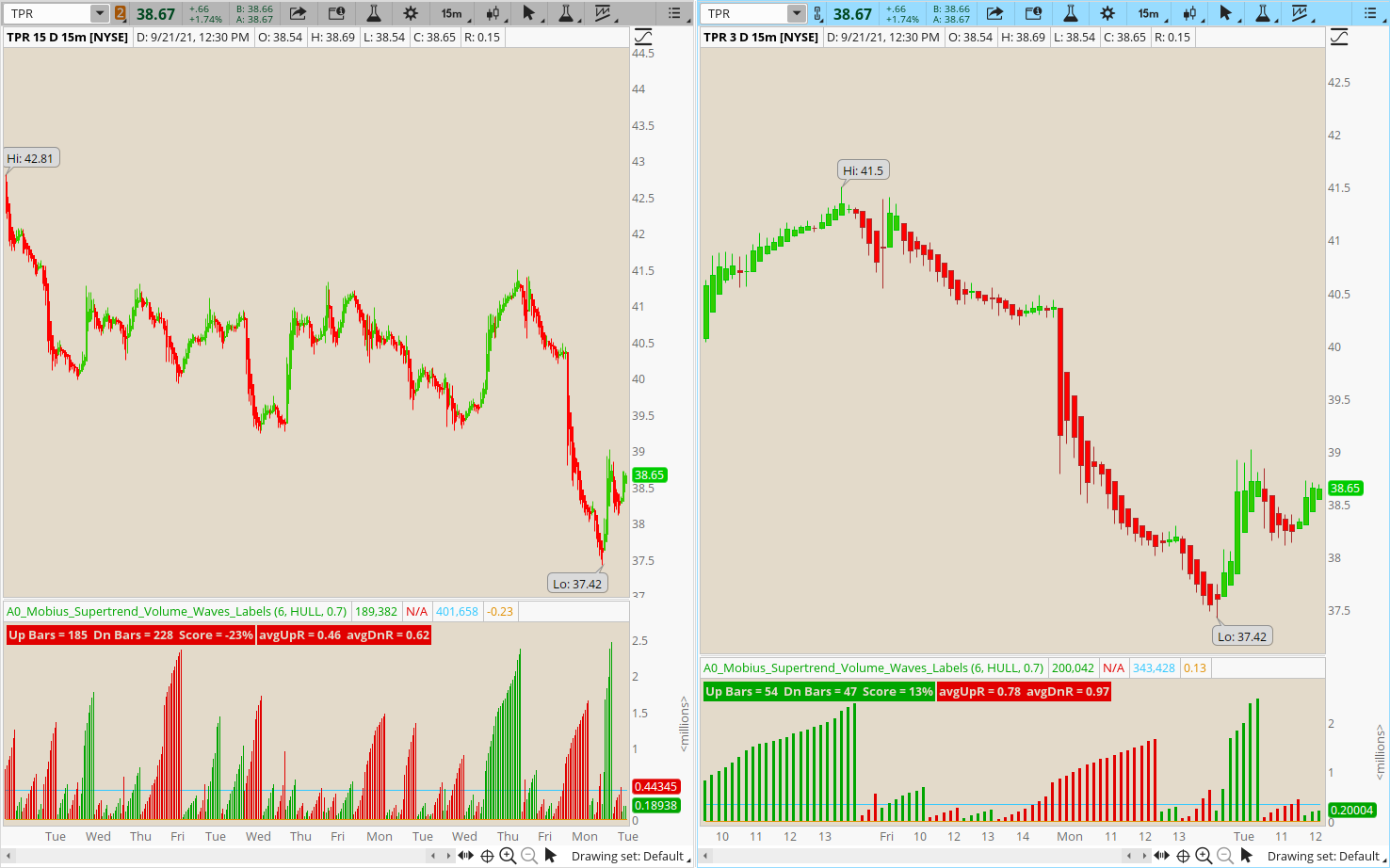Ruby:
``````# Mobius Volume Waves
# V01.2020
# Plots Volume Waves based on trend.

declare lower;

input ShowLabels = yes ;
input n = 6;
input AvgType = AverageType.HULL;
input ATRmult = .7;

def h = high;
def l = low;
def c = close;
def v = volume;
DefineGlobalColor("Ascending", CreateColor(0, 165, 0));
DefineGlobalColor("descending", CreateColor(225, 0, 0));
DefineGlobalColor("avg", CreateColor(50, 200, 255));
def ATR = MovingAverage(AvgType, TrueRange(h, c, l), n);
def DN = HL2 + (AtrMult * ATR);
def UP = HL2 + (-AtrMult * ATR);
def cond = if c < cond
then DN
else UP;
def accumulation = cond == UP;
def distribution = cond == DN;
def w1 = if accumulation and !accumulation
then v
else if accumulation
then w1 + v
else Double.NaN;
def w2 = if distribution and !distribution
then v
else if distribution
then w2 + v
else Double.NaN;
plot waves1 = w1;
waves1.SetPaintingStrategy(PaintingStrategy.HISTOGRAM);
waves1.SetDefaultColor(GlobalColor("Ascending"));
plot waves2 = w2;
waves2.SetPaintingStrategy(PaintingStrategy.HISTOGRAM);
waves2.SetDefaultColor(GlobalColor("Descending"));
def SD = StDevAll(v);
plot avg = (HighestAll(w1/2)+highestAll(w2/2))/n ;
avg.SetDefaultColor(GlobalColor("avg"));
def tsumUp = if accumulation
then tsumUp + 1
else tsumUp;
def tsumDn = if distribution
then tsumDn + 1
else tsumDn;
plot score = Round((tsumUp - tsumDn) / (tsumUp), 2);
AddLabel(ShowLabels, "Up Bars = " + tsumUp +
"  Dn Bars = " + tsumDn +
"  Score = " + AsPercent(score), if score > 0
then GlobalColor("Ascending")
else GlobalColor("descending"));
def upL;
def upH;
def upR;
def dnL;
def dnH;
def dnR;
if !IsNaN(w1) and !IsNaN(w2)
{
upL = l;
upH = upH;
upR = upR;
dnL = l;
dnH = dnH;
dnR = dnH - l;
}
else if !IsNaN(w2) and !IsNaN(w1)
{
upL = upL;
upH = h;
upR = h - upL;
dnL = dnL;
dnH = h;
dnR = dnR;
}
else
{
upL = upL;
upH = upH;
upR = upR;
dnL = dnL;
dnH = dnH;
dnR = dnR;
}
def tsumUpR = TotalSum(if upR != upR then upR else 0);
def tsumDnR = TotalSum(if dnR != dnR then dnR else 0);
def tsumW = TotalSum(!IsNaN(w1) and !IsNaN(w2));
def avgUpR = Round((tsumUpR / tsumW) / TickSize(), 0) * TickSize();
def avgDnR = Round((tsumDnR / tsumW) / TickSize(), 0) * TickSize();
AddLabel(ShowLabels , "avgUpR = " + avgUpR +
"  avgDnR = " + avgDnR, if avgUpR > avgDnR
then GlobalColor("Ascending")
else if avgUpR < avgDnR
then GlobalColor("descending")
else Color.blue);
# End Code Mobius Volume Waves``````

Last edited:
•Beltrame1

#### MerryDay

Staff member
Staff
VIP
Mobius Supertrend Volume Waves PLUS Welkin Volume Labels
Volume Waves present a simple visual picture. But I find I still want my volume statistics. So I added Welkin's Volume Statistics
Ruby:
``````# Mobius Supertrend Volume Waves PLUS Welkin Volume Labels
# V01.2020
# Plots Volume Waves based on trend.

declare lower;

input n = 6;
input AvgType = AverageType.HULL;
input ATRmult = .7;
def h = high;
def l = low;
def c = close;
def v = volume;

DefineGlobalColor("Ascending", CreateColor(0, 165, 0));
DefineGlobalColor("descending", CreateColor(225, 0, 0));
DefineGlobalColor("avg", Color.CYAN);
def ATR = MovingAverage(AvgType, TrueRange(h, c, l), n);
def DN = HL2 + (AtrMult * ATR);
def UP = HL2 + (-AtrMult * ATR);
def cond = if c < cond
then DN
else UP;
def accumulation = cond == UP;
def distribution = cond == DN;
def w1 = if accumulation and !accumulation
then v
else if accumulation
then w1 + v
else Double.NaN;
def w2 = if distribution and !distribution
then v
else if distribution
then w2 + v
else Double.NaN;

plot waves1 = w1;
waves1.SetPaintingStrategy(PaintingStrategy.HISTOGRAM);
waves1.SetDefaultColor(GlobalColor("Ascending"));
waves1.SetLineWeight(5);
plot waves2 = w2;
waves2.SetPaintingStrategy(PaintingStrategy.HISTOGRAM);
waves2.SetDefaultColor(GlobalColor("Descending"));
waves2.SetLineWeight(5);
def SD = StDevAll(v);
plot avg = (HighestAll(w1/2)+highestAll(w2/2))/n ;
avg.SetDefaultColor(GlobalColor("avg"));
avg.SetLineWeight(3);
# End Code

#[email protected]
#v5.22.2020

input AvgDayVolLength = 5;
input AvgVolLength = 20;
input ShowDayVolLabel = yes;
input ShowBarVolLabel = yes;

input VolAverageType = AverageType.SIMPLE;
input RelativetoPrevVolTolerance = 1.25; #if volume is 1.25x greater than previous bar it will paint even if it is still below the average/sigma2/sigma3

def NA = Double.NaN;
def PriceRange = high - low;
def TopShadowRange = if open >= close then high - open else high - close;
def BottomShadowRange = if open <= close then open - low else close - low;
def BodyRangeVolValue = ((BodyRange + BottomShadowRange) / PriceRange) * volume;
def DayVolAgg = if GetAggregationPeriod() < AggregationPeriod.DAY then AggregationPeriod.DAY else GetAggregationPeriod();

def DayVol = volume("period" = DayVolAgg);
def AvgDayVol = Average(DayVol, AvgDayVolLength);
def Start = 0930;
def End = 1600;
def conf = SecondsFromTime(Start) >= 0 and SecondsFromTime(End) <= 0;

plot VolColor = NA;
VolColor.DefineColor("Bullish", Color.GREEN);
VolColor.DefineColor("Bearish", Color.RED);
VolColor.DefineColor("VolAvg", CreateColor(0, 100, 200));
VolColor.DefineColor("VolSigma2", Color.DARK_ORANGE);
VolColor.DefineColor("VolSigma3", Color.MAGENTA);
VolColor.DefineColor("Relative to Prev", Color.YELLOW);
VolColor.DefineColor("Below Average", Color.GRAY);

#Current Candle Buy and Sell Strength
def BuyStr = ((close - low) / PriceRange) * 100;
def SellStr = ((high - close) / PriceRange) * 100;

def SellVol = (SellStr / 100) * volume;

def Vol = volume;
def VolAvg = MovingAverage(VolAverageType, volume, AvgVolLength);

#2Sigma and 3Sigma Vol Filter
def Num_Dev1 = 2.0;
def Num_Dev2 = 3.0;
def averageType = AverageType.SIMPLE;
def sDev = StDev(data = Vol, length = AvgVolLength);

def VolSigma2 = VolAvg + Num_Dev1 * sDev;
def VolSigma3 = VolAvg + Num_Dev2 * sDev;

def RelDayVol = DayVol / AvgDayVol;
def RelPrevDayVol = DayVol / volume("period" = DayVolAgg);
#Daily aggregation volume labels
AddLabel(if GetAggregationPeriod() >= AggregationPeriod.DAY then 0 else if ShowDayVolLabel then 1 else 0, "DayVol: " + DayVol + " / " + Round(RelDayVol, 2) + "x Avg(" + AvgDayVolLength + ") / " + Round(RelPrevDayVol, 2) + "x Prev", if DayVol > AvgDayVol then VolColor.Color("VolAvg") else VolColor.Color("Below Average"));

def RelVol = Vol / VolAvg;
def RelPrevVol = volume / volume;

#Triangle Vol Signal
def VolSignal = if Vol > VolSigma3 then volume else if Vol > VolSigma2 then volume else if Vol > VolAvg then volume else if RelPrevVol >= RelativetoPrevVolTolerance then volume else NA;

#current aggregation's volume labels
AddLabel(ShowBarVolLabel, "Vol: " + volume + " / " + Round(RelVol, 2) + "x Avg(" + AvgVolLength + ") / " + Round(RelPrevVol, 2) + "x Prev",  if Vol > VolSigma3 then VolColor.Color("VolSigma3") else if Vol > VolSigma2 then VolColor.Color("VolSigma2") else if Vol > VolAvg then VolColor.Color("VolAvg") else VolColor.Color("Below Average"));

DefineGlobalColor("LabelGreen",  CreateColor(0, 165, 0)) ;
AddLabel(if ShowBuySellStrength then 1 else 0, "Sell " + Round(SellStr, 2) + "%", if SellStr > BuyStr then Color.RED else Color.DARK_RED);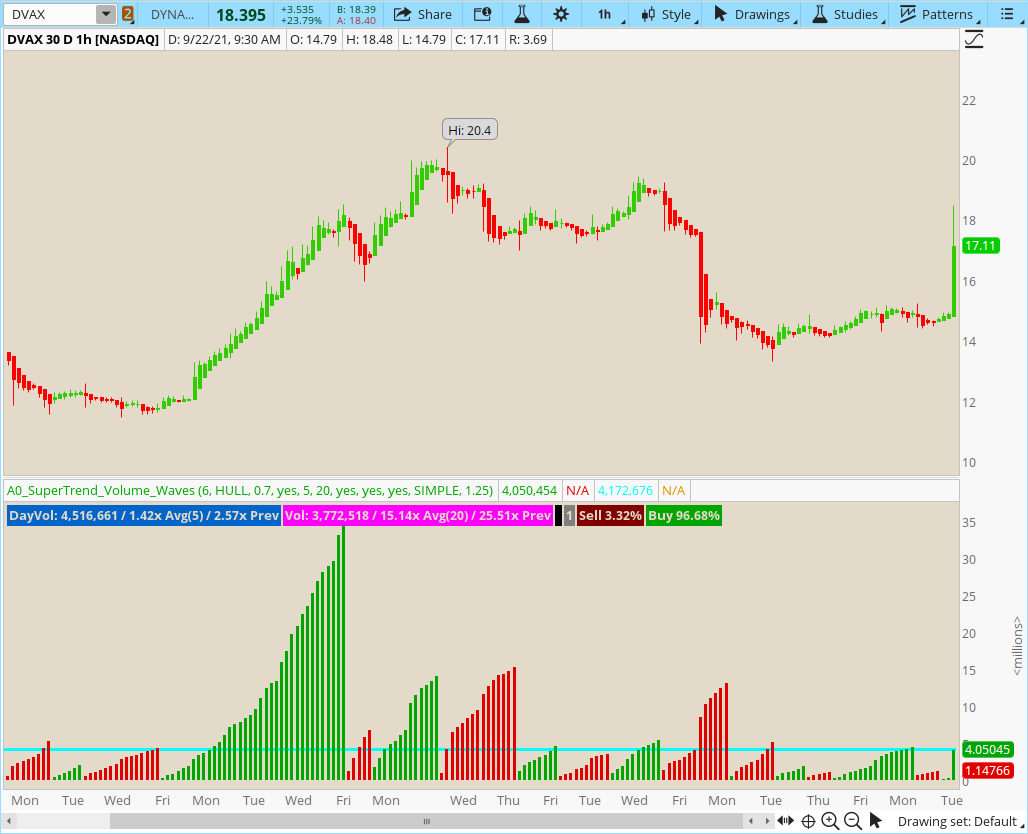Last edited:

##### Member
VIP
Mobius Supertrend Volume Waves also from on JohnnyQuotron's OneNote
View attachment 957

Ruby:
``````# Mobius Supertrend Volume Waves
# V01.2020
# Plots Volume Waves based on trend.

declare lower;

input n = 6;
input AvgType = AverageType.HULL;
input ATRmult = .7;
input ColorCandles = yes;
def h = high;
def l = low;
def c = close;
def v = volume;
DefineGlobalColor("Ascending", Color.GREEN);
DefineGlobalColor("descending", Color.RED);
DefineGlobalColor("avg", Color.CYAN);
def ATR = MovingAverage(AvgType, TrueRange(h, c, l), n);
def DN = HL2 + (AtrMult * ATR);
def UP = HL2 + (-AtrMult * ATR);
def cond = if c < cond
then DN
else UP;
def accumulation = cond == UP;
def distribution = cond == DN;
def w1 = if accumulation and !accumulation
then v
else if accumulation
then w1 + v
else Double.NaN;
def w2 = if distribution and !distribution
then v
else if distribution
then w2 + v
else Double.NaN;
plot waves1 = w1;
waves1.SetPaintingStrategy(PaintingStrategy.HISTOGRAM);
waves1.SetDefaultColor(GlobalColor("Ascending"));
plot waves2 = w2;
waves2.SetPaintingStrategy(PaintingStrategy.HISTOGRAM);
waves2.SetDefaultColor(GlobalColor("Descending"));
def SD = StDevAll(v);
plot avg = (HighestAll(w1/2)+highestAll(w2/2))/n ;
avg.SetDefaultColor(GlobalColor("avg"));
AssignPriceColor(if ColorCandles and cond == dn
then color.red
else color.green);
# End Code``````
Interesting study! Thanks! The Supertrend version I guess is to be used with the Supertrend indicator. Is it possible to scan for changes in the Volume Waves, when red changes to green and vice versa?

•DB01

#### MerryDay

Staff member
Staff
VIP
@Tradervic Not at all. Not related to the SuperTrend at all. Just the name that Mobius gave the indicator.
The Supertrend Volume Waves is the exact same as the Volume Waves except it has an additional plot:
plot avg = (HighestAll(w1/2)+highestAll(w2/2))/n ;​
It plots the highest average of the bearish and bullish waves. Waves that are above the average plot are super trending.

Thank you for pointing out the confusion. I will update the original post.

#### Mr_Wheeler

##### Active member
The labels are next to impossible for me to read when they turn red.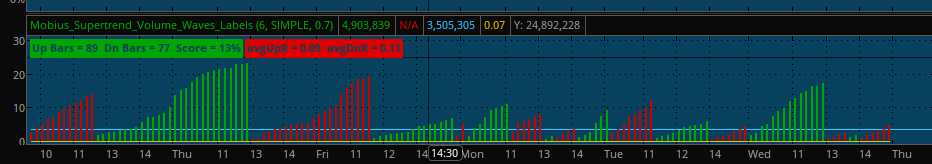#### Mr_Wheeler

##### Active member
To clarify things what does "at rmult" represent?

#### MerryDay

Staff member
Staff
VIP
@Mr_Wheeler
The AtrMult is used to define whether the volume is distributing or accumulating:
Code:
``````def DN = HL2 + (AtrMult * ATR);
def UP = HL2 + (-AtrMult * ATR);
def cond = if c < cond
then DN
else UP;
def accumulation = cond == UP;
def distribution = cond == DN;``````

#### Mr_Wheeler

##### Active member
@Mr_Wheeler
The AtrMult is used to define whether the volume is distributing or accumulating:
Code:
``````def DN = HL2 + (AtrMult * ATR);
def UP = HL2 + (-AtrMult * ATR);
def cond = if c < cond
then DN
else UP;
def accumulation = cond == UP;
def distribution = cond == DN;``````
By increasing value such as 0.7 to 0.8 I would be eliminating "noise?" I've got the figure up to "1.0" and I like it the way the study looks. I would just like to have you clarify to be sure that I don't have the wrong Pre-conception.

Would you please add a feature to remove the labels in the study's options? I don't really look at the label, and it's a nuisance, but it would be nice to be able to retain that feature.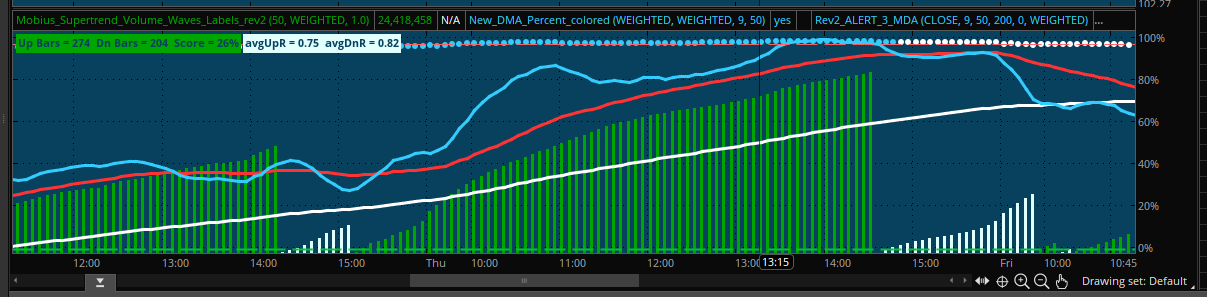https://tos.mx/kKdta1j

#### MerryDay

Staff member
Staff
VIP
@Mr_Wheeler post#3 has been updated with the option to not show labels.

While I will say that other Mobius studies start w/ a default of ATRmult equal to closer to 1, I am thinking there is a reason that it is so much lower on this indicator. I would recommend that you keep two versions on your charts. One w/ the default and one w/ your changes and observe it across history, across timeframes, across instruments, etc... Because making it so much higher than the default might have unforeseen effects.

#### Mr_Wheeler

##### Active member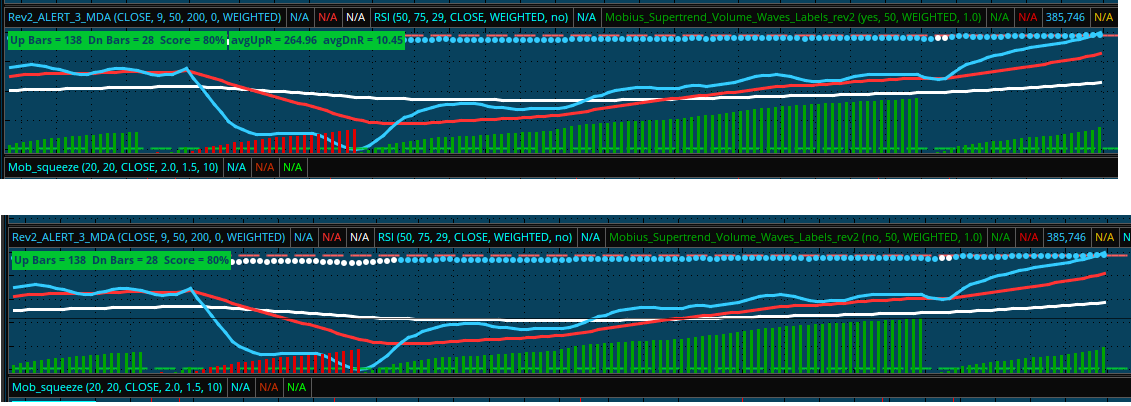Thanks for updating the study. Due this study in the combination of other studies as well I now know when to get out of trades. I'm now trying to figure out a combination studies to confirm enough momentum that warrants entering a trade.

It's one thing for the MDA lines to cross each other a little bit.

Last edited by a moderator:

#### Zlotko

##### Active member
VIP
By increasing value such as 0.7 to 0.8 I would be eliminating "noise?" I've got the figure up to "1.0" and I like it the way the study looks. I would just like to have you clarify to be sure that I don't have the wrong Pre-conception.

Would you please add a feature to remove the labels in the study's options? I don't really look at the label, and it's a nuisance, but it would be nice to be able to retain that feature.https://tos.mx/kKdta1j
How can I get the DMA % and 3-MDA on it/

moderator edit:

Last edited by a moderator:

#### Mr_Wheeler

##### Active member
•MerryDay

#### Mr_Wheeler

##### Active member
How can I get the DMA % and 3-MDA on it/

I've noticed that the top of that chart acts likes a oversold/over bought study. I've set up the percent study to use not lines but the "boolean" dots so it's easy to notice when a DMA cross over happens. With lines or bars it's some times difficult to tell if there was a cross over.

If the dots are blue I know that the 9 MDA has crossed the 50 MDA, and when the dots turn white I know that the trend has ended.

• -The 3 MDA study exists so that I don't have to constantly add three different MDA lines, and I'm able to configure them to a desirable look such as colors.

• The Blue dots. + The 9 MDA line touching the very top area. + the green mobius wave either vanishing or turning red means that I should get out of a trade.

• I like getting in a trade when there's blue dots with a green mobius wave starting to grow, if there are blue dots but a red wave under the blue dots then I avoid making a trade. Same goes for white dots and green wave, I avoid making a trade.

"mob squeeze" indicator - https://tos.mx/bbC3zMT

This is another indicator. I found it on this forum.

• I don't use it for it's original intent which is detecting short squeezes. I use it as another layer of trend confirming. Sometimes there's a tiny amount of bear volume that throws your Moving day averages off. If it has touched the line you know for sure that the trend has ended.

• I created a custom label study to remind me how to trade with all of my studies in order to not let my emotions get ahold of me.

• I configured all of my studies in the different panels to match up with the "weighted MDA average". I configured this "mob squeeze" indicator so that a dot would land directly on the line.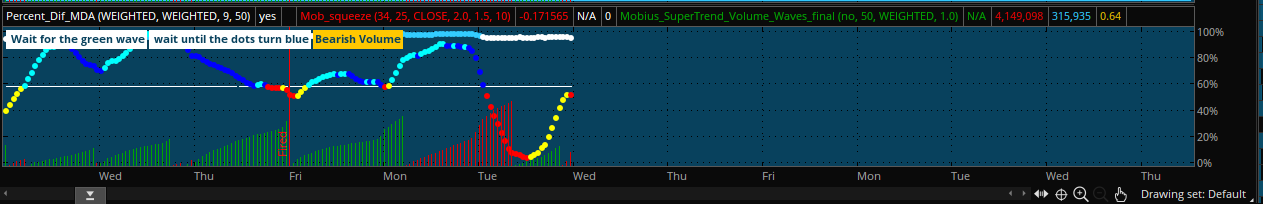Last edited:
•agirlhasnoname42 and MerryDay

#### RajB

##### Member
Mobius Supertrend Volume Waves also from on JohnnyQuotron's OneNote
This indicator has no correlation and it is not related AT ALL with the Mobius SuperTrend.​
The Supertrend Volume Waves is the exact same as the Volume Waves except it has an additional plot:

It plots the highest average of the bearish and bullish waves. Waves that are above the average plot are said to be on a super trend.

View attachment 957

Ruby:
``````# Mobius Supertrend Volume Waves
# V01.2020
# Plots Volume Waves based on trend.

declare lower;

input n = 6;
input AvgType = AverageType.HULL;
input ATRmult = .7;
input ColorCandles = no;
def h = high;
def l = low;
def c = close;
def v = volume;
DefineGlobalColor("Ascending", Color.GREEN);
DefineGlobalColor("descending", Color.RED);
DefineGlobalColor("avg", Color.CYAN);
def ATR = MovingAverage(AvgType, TrueRange(h, c, l), n);
def DN = HL2 + (AtrMult * ATR);
def UP = HL2 + (-AtrMult * ATR);
def cond = if c < cond
then DN
else UP;
def accumulation = cond == UP;
def distribution = cond == DN;
def w1 = if accumulation and !accumulation
then v
else if accumulation
then w1 + v
else Double.NaN;
def w2 = if distribution and !distribution
then v
else if distribution
then w2 + v
else Double.NaN;
plot waves1 = w1;
waves1.SetPaintingStrategy(PaintingStrategy.HISTOGRAM);
waves1.SetDefaultColor(GlobalColor("Ascending"));
plot waves2 = w2;
waves2.SetPaintingStrategy(PaintingStrategy.HISTOGRAM);
waves2.SetDefaultColor(GlobalColor("Descending"));
def SD = StDevAll(v);
plot avg = (HighestAll(w1/2)+highestAll(w2/2))/n ;
avg.SetDefaultColor(GlobalColor("avg"));
AssignPriceColor(if ColorCandles and cond == dn
then color.red
else color.green);
[INDENT]# End Code``````
[/INDENT]
@MerryDay
• found a minor issue in AssignPriceColor not honoring ColorCandles Input
• I modified my version as below, FYI
Ruby:
``````AssignPriceColor(if ColorCandles and cond == DN
then Color.RED
else if ColorCandles and !(cond == DN)
then Color.GREEN else Color.CURRENT);``````

•TheSpaniard

#### docsep

##### New member
Is there a scan for Volume wave? Or one for Percent_Dif_DMA?

#### Mr_Wheeler

##### Active member
Is there a scan for Volume wave? Or one for Percent_Dif_DMA?

The Percent_Dif_DMA simply tells one how big of a gap one DMA is away from another DMA line.

I configured it to use the "Boolean Point @ close" & set it up so that if the 9 MDA crosses the 50 MDA line I start to get blue dots, but when the 9 MDA crosses under the 50 MDA line the dots switch to a white color.

I choose the white color to represent a white surrender flag, and how it looks nice with my chart background color.

My scanner setting that I'm actively tweaking - https://tos.mx/PNKPm1Y

I'm trying to set it up so that it spits out stocks that have a 9 MDA line under the 50 MDA which is under 200 MDA. My current scanner setting might be the one I stick with.

------------------------------------

Here's a problem that I've run into. The Scanner software lies to you.

I've set up scanner tab to display the 9,50, and 200 weighted MDA line values.

What i've found is that if you were filter for stocks that have their 50 MDA under the 200 MDA, and their 9 MDA line is under the 50 MDA line, then when you check the stock out you'll find that in reality the stock has the 50 MDA above the 200 MDA line, and the 9 mda line above the 50 MDA & the stock is over sold.

The scanner lied with the "Percent_Dif_DMA" as well. The value listed on the scanner was completely different than the "percent_Dif_DMA" value on the chart.

This is my current chart - https://tos.mx/PNKPm1Y

My current chart doesn't have a "percent_Dif_DMA" column, but I was able to add one without no problem..

You click on the icon in the orange box to create the "percent_Dif_DMA" column.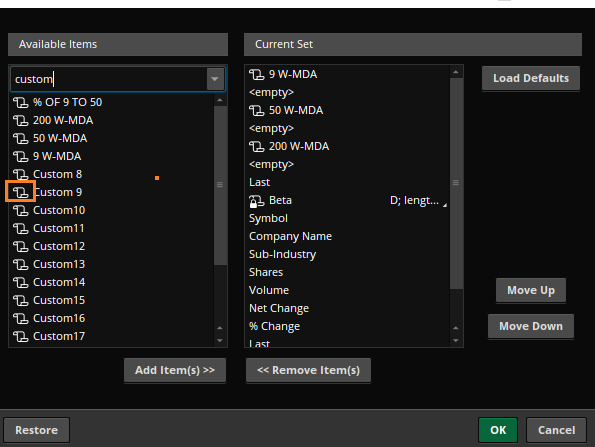•docsep

#### docsep

##### New member
The Percent_Dif_DMA simply tells one how big of a gap one DMA is away from another DMA line.

I configured it to use the "Boolean Point @ close" & set it up so that if the 9 MDA crosses the 50 MDA line I start to get blue dots, but when the 9 MDA crosses under the 50 MDA line the dots switch to a white color.

I choose the white color to represent a white surrender flag, and how it looks nice with my chart background color.

My scanner setting that I'm actively tweaking - https://tos.mx/PNKPm1Y

I'm trying to set it up so that it spits out stocks that have a 9 MDA line under the 50 MDA which is under 200 MDA. My current scanner setting might be the one I stick with.

------------------------------------

Here's a problem that I've run into. The Scanner software lies to you.

I've set up scanner tab to display the 9,50, and 200 weighted MDA line values.

What i've found is that if you were filter for stocks that have their 50 MDA under the 200 MDA, and their 9 MDA line is under the 50 MDA line, then when you check the stock out you'll find that in reality the stock has the 50 MDA above the 200 MDA line, and the 9 mda line above the 50 MDA & the stock is over sold.

The scanner lied with the "Percent_Dif_DMA" as well. The value listed on the scanner was completely different than the "percent_Dif_DMA" value on the chart.

This is my current chart - https://tos.mx/PNKPm1Y

My current chart doesn't have a "percent_Dif_DMA" column, but I was able to add one without no problem..

You click on the icon in the orange box to create the "percent_Dif_DMA" column.Thank you

#### gate122

##### New member
I've noticed that the top of that chart acts likes a oversold/over bought study. I've set up the percent study to use not lines but the "boolean" dots so it's easy to notice when a DMA cross over happens. With lines or bars it's some times difficult to tell if there was a cross over.

If the dots are blue I know that the 9 MDA has crossed the 50 MDA, and when the dots turn white I know that the trend has ended.

• -The 3 MDA study exists so that I don't have to constantly add three different MDA lines, and I'm able to configure them to a desirable look such as colors.

• The Blue dots. + The 9 MDA line touching the very top area. + the green mobius wave either vanishing or turning red means that I should get out of a trade.

• I like getting in a trade when there's blue dots with a green mobius wave starting to grow, if there are blue dots but a red wave under the blue dots then I avoid making a trade. Same goes for white dots and green wave, I avoid making a trade.

"mob squeeze" indicator - https://tos.mx/bbC3zMT

This is another indicator. I found it on this forum.

• I don't use it for it's original intent which is detecting short squeezes. I use it as another layer of trend confirming. Sometimes there's a tiny amount of bear volume that throws your Moving day averages off. If it has touched the line you know for sure that the trend has ended.

• I created a custom label study to remind me how to trade with all of my studies in order to not let my emotions get ahold of me.

• I configured all of my studies in the different panels to match up with the "weighted MDA average". I configured this "mob squeeze" indicator so that a dot would land directly on the line.Do you mind sharing me your setup as I am having a hard time trying to match it base on your screenshot. Love to test what you have. Thanks.

### Not the exact question you're looking for?

87k+ Posts
290 Online## The Market Trading Game Changer

Join 2,500+ subscribers inside the useThinkScript VIP Membership Club
• Exclusive indicators
• Proven strategies & setups
• Private Discord community
• Exclusive members-only content
• 1 full year of unlimited support

What is useThinkScript?

useThinkScript is the #1 community of stock market investors using indicators and other tools to power their trading strategies. Traders of all skill levels use our forums to learn about scripting and indicators, help each other, and discover new ways to gain an edge in the markets.

How do I get started?

We get it. Our forum can be intimidating, if not overwhelming. With thousands of topics, tens of thousands of posts, our community has created an incredibly deep knowledge base for stock traders. No one can ever exhaust every resource provided on our site.

If you are new, or just looking for guidance, here are some helpful links to get you started.

What are the benefits of VIP Membership?